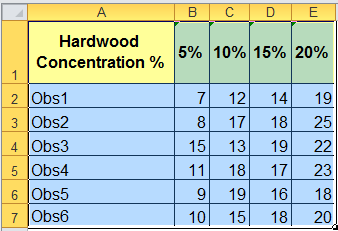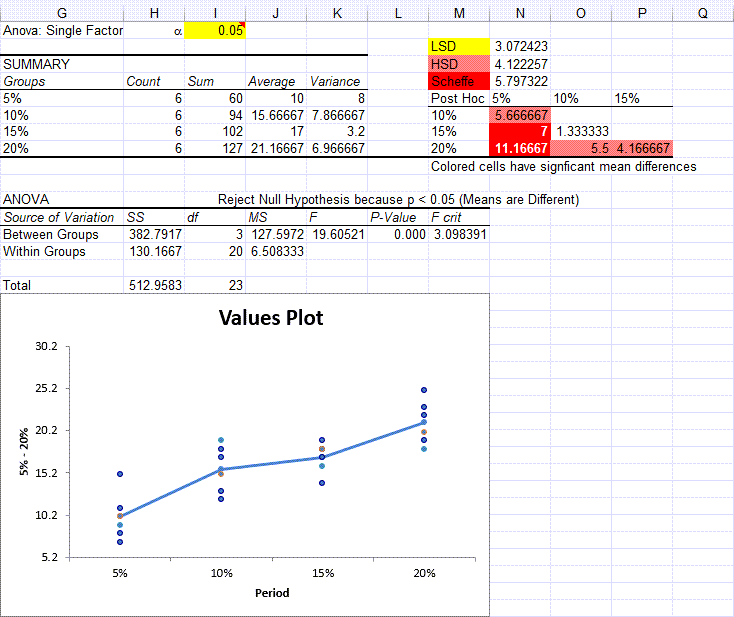# Need to Run One-Way ANOVA Post Hoc Tests in Excel?

## QI Macros runs Post Hoc tests in Excel. Excel's data analysis tool doesn't.

#### To run one-way ANOVA using QI Macros

2. Click on QI Macros menu > Statistical Tools > ANOVA > ANOVA Single Factor.
3. QI Macros will do the math and analysis for you.

After a one-way ANOVA finds a significant difference in means, Post Hoc testing helps identify which of the differences are significant.

### Three Common Post Hoc Tests

Post Hoc tests compare the differences between the means with various factors to identify significant differences. There are a number of ways that these factors are determined, but LSD, HSD and Scheffe's are three of the more common ones:

• LSD - Fisher's Least Significant Difference
• HSD - Tukey's Honestly Significant Difference reduces Type 1 error.
• Scheffe's is the most conservative of the three

Note: Excel does not do post hoc tests; QI Macros adds this functionality.

### Post hoc example using QI Macros and data from Montgomery's Intro to SPC book

Using the tensile strength data in columns B to E,QI Macros calculations are shown in columns G to P. The Post Hoc tests are in cells M2:P8.### Interpretation of Results

QI Macros built in code compares the p-value (it calculates) to the significance level (you input) to tell you what the results mean. You will see one of two results:

• Reject the null hypothesis - Means are different/Means are not the same.
• Cannot Reject the null hypothesis (Accept the null hypothesis) - Means are the same/Means are not different

In this example, the p-value is less than the significance level 0.05, so the Means are considered Different. If the mean difference is greater than LSD, HSD or Scheffe's, the difference is considered significant.

#### QI Macros uses color coding to help you understand the post hoc tests.

Since Scheffe > HSD > LSD, QI Macros identifies significant differences by shading the results as follows:

• greater than Scheffe's it is shown in red
• less than Scheffe but greater than HSD it is shown in rose
• less than HSD but greater than LSD, it is shown in yellow
• less than LSD, no shading

In the example below the difference between these tensile strengths is:

• greater than Scheffe (5.797) so they are shaded in red: 5% and 15%, 5% and 20%
• less than Scheffe (5.797) but greater than HSD (4.122) so they are shaded in rose: 5% and 10%, 10% and 20%, 15% and 20%
• less than HSD (4.122) but greater than LSD (3.072) so they are shaded in yellow: none
• less than LSD (3.072) so they are not shaded at all: 10% and 15%### Let QI Macros do the work for you.

QI Macros is the only SPC software that compares the p-value (0.000) to the significance (0.05) and tells you to "Reject the Null Hypothesis because p<0.05" and that the "Means are Different/Means are not the Same". Then the Post Hoc tests tell you which differences are significant.